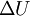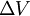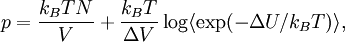Test volume method

(diff) ← Older revision | Latest revision (diff) | Newer revision → (diff)
An alternative to the virial pressure route to calculating the pressure, there is a method which consists on evaluating the change in internal energy,$\Delta U$ produced by a small change in the volume of the system$\Delta V$. It can be shown that$p = \frac{ k_B T N}{V} + \frac{ k_B T }{ \Delta V } \log \langle \exp(-\Delta U/k_B T )\rangle,$
where$k_B$ is the Boltzmann constant and$T$ is the temperature.